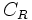# Elastic collision

(Redirected from Perfectly elastic collision)

## Definition

A collision between two bodies is termed an elastic collision or perfectly elastic collision if it satisfies the following equivalent conditions:

1. The total kinetic energy of the bodies after the collision equals the total kinetic enery of the bodies before the collision.
2. The coefficient of restitution of the collision is$1$, i.e., the velocity difference between the bodies after collision is equal in magnitude and opposite in sign to the velocity difference before the collision (this is the definition for head-on collisions; for oblique collisions, we consider the components of velocity in the normal direction).

Elastic collisions are an ideal approximation that may not occur in practice, hence the term perfectly elastic is sometimes used to describe such collisions.

## Related notions

• Coefficient of restitution: Usually denoted$e$ or$C_R$, this number, which typically varies between$0$ and$1$, measures the bounciness of a collision. An elastic collision has coefficient of restitution equal to$1$, and a perfectly inelastic collision has a coefficient of restitution equal to$0$.
• Perfectly inelastic collision: A collision after which the two bodies stick to each other, i.e., their relative position remains the same as their relative position at the time of the collision.